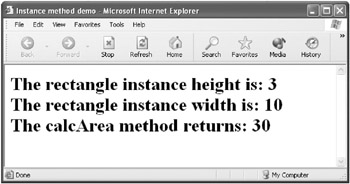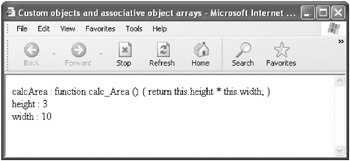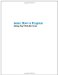# Creating Objects

 Advanced The first section in this chapter, “ Understanding Object-Oriented Programming,” explained OO in a fairly language-independent fashion. (The dot operator (.) discussed at the beginning of that section and used to reference a value associated with an object is pretty much universal across programming languages.) The next section, “ JavaScript OO Lite,” explained how to work with the objects that are exposed by an HTML document in a Web browser. I also showed you the objects that are provided by the JavaScript programming language.

It’s time to turn to the task of creating custom objects in JavaScript that use some of the OO concepts I’ve explained so far.

The most crucial issue is to understand the role of prototypes, which are the JavaScript analog to classes and which play the same role as classes in providing a template for objects.

The main limitation of a JavaScript prototype as opposed to the more general concept of a class is that only one generation of inheritance is available with a prototype. With a prototype, you create many instances of objects based on the prototype. But with classes, you can have one class that inherits from another class that inherits from another class (and so on), finally creating instances of objects from the class that’s the remote descendent of the original class. This can be very powerful. But other than the ability to inherit multiple generations from a blueprint, you should think of a JavaScript prototype as a class.

I’ll explain how prototypes work as we go along. But, first, let’s look at two JavaScript keywords related to objects, new and this. The new keyword, which you’ve seen used in many examples in earlier chapters of this book (for example, you used new to create instances of Array objects in Chapter 5, “Understanding Arrays”), calls a constructor to create a new instance of an object.

The this keyword is used to refer to the current instance of an object.

 Note The keywords new and this are used quite widely in OO languages for the same purposes as in JavaScript although there are some variations. For example, me is used instead of this in Visual Basic .NET.

As I’ve indicated, JavaScript has no explicit concept of a class, which makes it less than an ideal choice as a language to teach OO. But please keep the general concept of a class in mind as we go over what JavaScript does have, namely, prototype objects. Each object has a prototype object, and an object inherits all the members of its prototype. By inherits, I mean that all the members defined for the prototype can be found in any instance object that’s based on the prototype. So in this way, a prototype object functions exactly like a class. The prototype object is the blueprint, or cookie cutter, and all the instance objects based upon it are the buildings (or cookies).

By the way, whether you’re talking classes or prototypes, it’s conventional to name a class (or prototype) starting with an initial capital letter. Objects instantiated from the class (or prototype) are named with an initial lowercase letter. For example, you could have a Car class and an object based on it named theJaguar.

Let’s look at an example that demonstrates some of these concepts using JavaScript code. Because this is our first example that shows how to create and use our own custom objects in JavaScript, what we’ll do will be relatively simple so that you can see the bare-bones concepts at work. Our task will be to create a Rectangle prototype. The Rectangle prototype will have a constructor function that takes as arguments the height and width of a rectangle instance. You can also set height and width values as properties of rectangle instances. Finally, the Rectangle prototype will supply a method, calcArea, that calculates the area of a given rectangle instance.

Here goes!

Let’s start with the constructor function that’s used to create a Rectangle object. As noted, it accepts two arguments, one for the height and the other for the width of the instance of the Rectangle object. Within the constructor function, the this keyword is used to assign the value passed to the function to the instance property:

` function Rectangle(height, width){     this.height =  height;     this.width = width;  } `

Next, we’ll create the function that will be used to implement the calcArea method:

` function calc_Area () {     return this.height * this.width;  } `

This function returns the value of the instance height multiplied by the instance width. Note that I’ve named it slightly differently than the method (calc_Area rather than calcArea). This is to emphasize that the name used to call the method, using an instance of the prototype, is distinct from the underlying function that implements the method.

Now, let’s assign the function we’ve just created to the value of the Rectangle prototype, using the prototype keyword, so that we can call it as an instance method:

` Rectangle.prototype.calcArea = calc_Area; `

Our simple Rectangle prototype is now complete. Let’s instantiate an object, named theRectangle, based upon it:

` var theRectangle = new Rectangle (3, 5); `

To show we can, let’s set a property value of the instantiated theRectangle object (replacing the original value of five set in the constructor when theRectangle was instantiated):

` theRectangle.width = 10; `

Finally, we can display theRectangle object’s current property values and call its calcArea method to display its area as shown in Figure 7-11:

` document.write("The rectangle instance height is: " +     theRectangle.height + "<br>");  document.write("The rectangle instance width is: " + theRectangle.width  +  "<br>");  document.write ("The calcArea method returns: " +  theRectangle.calcArea()); `Figure 7-11: The object instance’s values of its height and width properties and the return value for its calcArea method are displayed in the browser.

Listing 7-7 shows the complete code for creating the Rectangle prototype, instantiating theRectangle object based on the prototype, displaying property values, and invoking an object method.

Listing 7.7: Creating an Object and Using Object Instance Properties and Methods` <HTML> <HEAD> <TITLE>Instance method demo</TITLE> </HEAD> <BODY> <H1> <SCRIPT>     function Rectangle(height, width){     // constructor function        this.height =  height;        this.width = width;     }     // create the function     function calc_Area () {        return this.height * this.width;     }     // turn the function into an object method     Rectangle.prototype.calcArea = calc_Area;     // instantiate the object     var theRectangle = new Rectangle (3, 5);     // set an instance property     theRectangle.width = 10;     // call and display the instance properties and method return     document.write("The rectangle instance height is: " +        theRectangle.height + "<br>");     document.write("The rectangle instance width is: " +        theRectangle.width  + "<br>");     document.write ("The calcArea method returns: " +  theRectangle.calcArea());     </SCRIPT> </H1> </BODY> </HTML> `Earlier in this chapter I explained to you the concept of shared members of a class. The code in Listing 7-7 showed how to work with instance members of a class (or, more accurately, because we’re inhabiting JavaScript land, instance members of a prototype). An instance member requires that an object be instantiated, and it can have different values for each object based on a prototype (or class).

As opposed to instance members, to access a shared member you’re neither required (nor can) instantiate an object instance. The best way of thinking of shared members is that they’re a kind of constant value. You can also think of them as class (or prototype) variables rather than instance variables. Here are two examples of shared members in JavaScript using our Rectangle prototype:

` Rectangle.frodo = 12;  Rectangle.Pi = 3.1415; `

Here is an assignment statement that uses these shared members (note that no instantiation of a Rectangle object is required):

` var theRing = Rectangle.Pi * Rectangle.frodo * Rectangle.frodo; `

### Custom Objects As Arrays

In the “ Displaying an Object’s Associative Array ” section earlier in this chapter, I showed you how to display the array associated with the window object and other objects such as document and navigator.

You can just as easily display the members of your own objects using their associative arrays. As an example, take the Rectangle prototype and instance objects created in the previous section. If you pass the instance object to the function I showed you for displaying the elements of an object’s associative array, you’ll see the method and properties of the instance of the Rectangle object displayed, as shown in Figure 7-12.Figure 7-12: The properties of a Rectangle instance shown using its associative array

 Tip Because there’s no way to know the order a for/in statement will go through an associative array, when you run this program, your display may not match that shown in Figure 7-12.

Listing 7-8 shows the HTML page that defines the Rectangle object prototype, creates an object instance based upon it, and uses the for/in statement to iterate through the associative array of the object instance.

Listing 7.8: The Associative Array of a Rectangle Object Instance` <HTML> <HEAD> <TITLE>Custom objects and associative object arrays</TITLE> <SCRIPT>  function showProperties (theObject){     for (i in theObject) {        if (theObject[i] != null) {            document.write(i + " : " + theObject[i] + "<br>");        }        else {           document.write(i + "<br>");        }     }     return;  }  </SCRIPT> </HEAD> <BODY> <SCRIPT>  function Rectangle(height, width){     // constructor function        this.height = height;        this.width = width;     }     // create the function     function calc_Area () {        return this.height * this.width;     }     // turn the function into an object method     Rectangle.prototype.calcArea = calc_Area;     // instantiate the object     var theRectangle = new Rectangle (3, 5);     // set an instance property     theRectangle.width = 10;  showProperties (theRectangle);  </SCRIPT> </BODY> </HTML> `### Defining a Custom toString Method

As I mentioned earlier, it’s a good idea to define a custom toString method for your own objects. This custom toString method will be used when JavaScript needs to convert an instance of your object to a string type— often for the purpose of displaying a string representation of your object instance.

It’s easy to add a custom toString method to your objects. In fact, it works just like adding any other method to the prototype for object instances.

Let’s take our Rectangle prototype and object instance as an example to see how to do this.

First, write a new function that returns a string. The function can be named anything, but usually it should be named to show that it’s related to the toString method. The function that implements a toString method for a prototype will often use instance values of objects based on the prototype when generating a return value.

Here’s my somewhat silly function for objects based on the Rectangle prototype:

` function to_String() {     return "I am a funny rectangle short and stout: " +         this.height + " by " +         this.width + "."  } `

Next, assign the function that implements the toString (to_String) method to the toString method of the prototype object:

` Rectangle.prototype.toString = to_String; `

Instantiate an object based on the prototype:

` var theRectangle = new Rectangle (2, 42); `

And finally, write code that invokes the toString method of the Rectangle prototype on the instance of the Rectangle object and display it (the results are shown in Figure 7-13).Figure 7-13: The custom toString method of the Rectangle object returns some cute text.

` document.write(theRectangle); `

This is kind of like magic, almost spooky. The statement document.write(theRectangle); would look like something that shouldn’t give intelligible results. (In fact, what you get without the custom toString method implementation is [object Object], which isn’t indeed very intelligible.)

By adding the toString method to the Rectangle prototype, coders can then casually involve instances of the Rectangle object in string conversions—and get reasonable results without worrying about it. Listing 7-9 shows an appropriate implementation of a toString method for the Rectangle object.

Listing 7.10: Implementing a toString Method` <HTML> <HEAD> <TITLE>Instance method demo</TITLE> </HEAD> <BODY> <H1> <SCRIPT>     function Rectangle(height, width){     // constructor function        this.height =  height;        this.width = width;     }     function calc_Area () {        return this.height * this.width;     }     function to_String() {        return "I am a funny rectangle short and stout: " +            this.height + " by " +            this.width + "."     }     Rectangle.prototype.calcArea = calc_Area;     Rectangle.prototype.toString = to_String;     var theRectangle = new Rectangle (2, 42);     document.write(theRectangle);     </SCRIPT> </H1> </BODY> </HTML> `Learn How to Program Using Any Web Browser
ISBN: 1590591135
EAN: 2147483647
Year: 2006
Pages: 115
Authors: Harold Davis

Similar book on Amazon